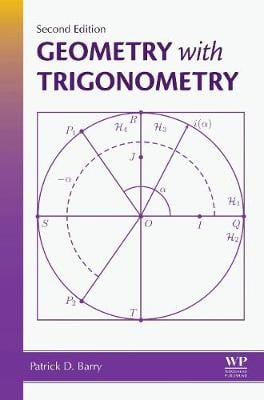•# Geometry with Trigonometry (Hardback)

(author)
£60.99
Hardback 280 Pages / Published: 26/11/2015
• We can order this

Usually dispatched within 3 weeks

Geometry with Trigonometry Second Edition is a second course in plane Euclidean geometry, second in the sense that many of its basic concepts will have been dealt with at school, less precisely. It gets underway with a large section of pure geometry in Chapters 2 to 5 inclusive, in which many familiar results are efficiently proved, although the logical frame work is not traditional. In Chapter 6 there is a convenient introduction of coordinate geometry in which the only use of angles is to handle the perpendicularity or parallelism of lines. Cartesian equations and parametric equations of a line are developed and there are several applications. In Chapter 7 basic properties of circles are developed, the mid-line of an angle-support, and sensed distances. In the short Chaper 8 there is a treatment of translations, axial symmetries and more generally isometries. In Chapter 9 trigonometry is dealt with in an original way which e.g. allows concepts such as clockwise and anticlockwise to be handled in a way which is not purely visual. By the stage of Chapter 9 we have a context in which calculus can be developed. In Chapter 10 the use of complex numbers as coordinates is introduced and the great conveniences this notation allows are systematically exploited. Many and varied topics are dealt with , including sensed angles, sensed area of a triangle, angles between lines as opposed to angles between co-initial half-lines (duo-angles). In Chapter 11 various convenient methods of proving geometrical results are established, position vectors, areal coordinates, an original concept mobile coordinates. In Chapter 12 trigonometric functions in the context of calculus are treated. New to this edition: The second edition has been comprehensively revised over three years Errors have been corrected and some proofs marginally improved The substantial difference is that Chapter 11 has been significantly extended, particularly the role of mobile coordinates, and a more thorough account of the material is given

Publisher: Elsevier Science Publishing Co Inc
ISBN: 9780128050668
Number of pages: 280
Weight: 420 g
Dimensions: 229 x 152 x 19 mm
Edition: 2nd edition